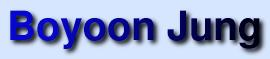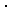Boyoon Jung's Library Manual

bj::EMCluster Class Reference [EM-based Clustering]

A model-based clustering algorithm using Expectation-Maximization method. More...

#include <em_cluster.h>

Inherited by bj::SMEMCluster.

Inheritance diagram for bj::EMCluster:[legend]
Collaboration diagram for bj::EMCluster:[legend]
List of all members.

Public Member Functions

EMCluster (int dimension, int max_data, gsl_vector *min=0, gsl_vector *max=0, int max_clusters=10, int max_iteration=10)
A constructor.
~EMCluster (void)
A destructor.
void initialize (double *pi=0, gsl_vector **mean=0, gsl_matrix **cov=0)
Initialize EM cluster.
virtual int cluster (gsl_vector **data, int size)
Cluster data points using gaussian mixture.
int numClusters (void)
Return the number clusters.
double weight (int c)
Return the weights of a specific cluster.
gsl_vector * mean (int c)
Return the mean vector of a specific cluster.
gsl_matrix * covariance (int c)
Return the covariance matrix of a specific cluster.
void setTerminationParams (double prt=0.0, double pat=1.e-8, double mrt=0.0, double mat=1.e-5, double crt=0.0, double cat=1.e-5)
Set the tolerance values for convergence test.

Static Public Member Functions

void cov2ellipse (gsl_matrix *cov, double &w, double &h, double &a)
Convert a covariance matrix to an ellipsoidal parameters.

Protected Member Functions

bool similar (double x, double y)
Check if two scalar values are similar enough.
bool similar (gsl_vector *x, gsl_vector *y)
Check if two vectors are similar enough.
bool similar (gsl_matrix *x, gsl_matrix *y)
Check if two matrices are similar enough.

Protected Attributes

int nclusters
Number of clusters.
double * pi
Weights (mixing proportion).
gsl_vector ** _mean
Mean vectors.
gsl_matrix ** cov
Covariance matrices.
double prev_pi
Previous weight for convergence test.
gsl_vector * prev_mean
Previous mean vector for convergence test.
gsl_matrix * prev_cov
Previous covariance matrix for convergence test.
double pi_rtol
Relative tolerance for convergence test.
double pi_atol
Absolute tolerance for convergence test.
double mean_rtol
Relative tolerance for convergence test.
double mean_atol
Absolute tolerance for convergence test.
double cov_rtol
Relative tolerance for convergence test.
double cov_atol
Absolute tolerance for convergence test.
double ** likelihood
Likelihoods.
double ** z
Hidden variables.
int dimension
The dimension of data.
int max_data
Maximum number of data.
gsl_vector * ds_min
Lower bound of the data space.
gsl_vector * ds_max
Upper bound of the data space.
int max_clusters
Maximum number of clusters.
int max_iteration
Maximum number of iteration.
MultivariateGaussiangaussian
Multivariate gaussian distribution.
gsl_rng * rng
Random number generator.
gsl_vector * tzx
Temporary buffer.
gsl_vector * td
Temporary buffer.

Detailed Description

A model-based clustering algorithm using Expectation-Maximization method.

EMCluster class implements a model-based data clustering algorithm using EM method. This class clusters a set of data points into a gaussian mixture.

The current implementation does not utilize the properties of covariance matrix (i.g. symmetric, positive-definite, etc.) for efficiency.

Constructor & Destructor Documentation

 bj::EMCluster::EMCluster ( int dimension, int max_data, gsl_vector * min = 0, gsl_vector * max = 0, int max_clusters = 10, int max_iteration = 10 )

A constructor.

Parameters:
 dimension The dimension of data points. max_data The maximum number of data points. min The lower bound of the data space. max The upper bound of the data space. max_clusters The maximum number of clusters. max_iteration The upper bound of iteration.

 bj::EMCluster::~EMCluster ( void )
 A destructor.

Member Function Documentation

 virtual int bj::EMCluster::cluster ( gsl_vector ** data, int size ) [virtual]
 Cluster data points using gaussian mixture. Returns:The number of iteration. Reimplemented in bj::SMEMCluster.

 void bj::EMCluster::cov2ellipse ( gsl_matrix * cov, double & w, double & h, double & a ) [inline, static]

Convert a covariance matrix to an ellipsoidal parameters.

Parameters:
 cov A covariance matrix. [out] w The width of an ellipsoidal. [out] h The height of an ellipsoidal. [out] a The angle (in radians) of an ellipsoidal.

 gsl_matrix* bj::EMCluster::covariance ( int c ) [inline]
 Return the covariance matrix of a specific cluster.

 void bj::EMCluster::initialize ( double * pi = 0, gsl_vector ** mean = 0, gsl_matrix ** cov = 0 )
 Initialize EM cluster.

 gsl_vector* bj::EMCluster::mean ( int c ) [inline]
 Return the mean vector of a specific cluster.

 int bj::EMCluster::numClusters ( void ) [inline]
 Return the number clusters.

 void bj::EMCluster::setTerminationParams ( double prt = 0.0, double pat = 1.e-8, double mrt = 0.0, double mat = 1.e-5, double crt = 0.0, double cat = 1.e-5 ) [inline]
 Set the tolerance values for convergence test.

 bool bj::EMCluster::similar ( gsl_matrix * x, gsl_matrix * y ) [inline, protected]
 Check if two matrices are similar enough.

 bool bj::EMCluster::similar ( gsl_vector * x, gsl_vector * y ) [inline, protected]
 Check if two vectors are similar enough.

 bool bj::EMCluster::similar ( double x, double y ) [inline, protected]
 Check if two scalar values are similar enough.

 double bj::EMCluster::weight ( int c ) [inline]
 Return the weights of a specific cluster.

Member Data Documentation

 gsl_vector** bj::EMCluster::_mean [protected]
 Mean vectors.

 gsl_matrix** bj::EMCluster::cov [protected]
 Covariance matrices.

 double bj::EMCluster::cov_atol [protected]
 Absolute tolerance for convergence test.

 double bj::EMCluster::cov_rtol [protected]
 Relative tolerance for convergence test.

 int bj::EMCluster::dimension [protected]
 The dimension of data.

 gsl_vector* bj::EMCluster::ds_max [protected]
 Upper bound of the data space.

 gsl_vector* bj::EMCluster::ds_min [protected]
 Lower bound of the data space.

 MultivariateGaussian* bj::EMCluster::gaussian [protected]
 Multivariate gaussian distribution.

 double** bj::EMCluster::likelihood [protected]
 Likelihoods.

 int bj::EMCluster::max_clusters [protected]
 Maximum number of clusters.

 int bj::EMCluster::max_data [protected]
 Maximum number of data.

 int bj::EMCluster::max_iteration [protected]
 Maximum number of iteration.

 double bj::EMCluster::mean_atol [protected]
 Absolute tolerance for convergence test.

 double bj::EMCluster::mean_rtol [protected]
 Relative tolerance for convergence test.

 int bj::EMCluster::nclusters [protected]
 Number of clusters.

 double* bj::EMCluster::pi [protected]
 Weights (mixing proportion).

 double bj::EMCluster::pi_atol [protected]
 Absolute tolerance for convergence test.

 double bj::EMCluster::pi_rtol [protected]
 Relative tolerance for convergence test.

 gsl_matrix* bj::EMCluster::prev_cov [protected]
 Previous covariance matrix for convergence test.

 gsl_vector* bj::EMCluster::prev_mean [protected]
 Previous mean vector for convergence test.

 double bj::EMCluster::prev_pi [protected]
 Previous weight for convergence test.

 gsl_rng* bj::EMCluster::rng [protected]
 Random number generator.

 gsl_vector* bj::EMCluster::td [protected]
 Temporary buffer.

 gsl_vector* bj::EMCluster::tzx [protected]
 Temporary buffer.

 double** bj::EMCluster::z [protected]
 Hidden variables.

The documentation for this class was generated from the following file:
ResearchProjectsPublications

ToolsBjLib ManualPlayer/StagePyPlayerMicroStrain IMUGPS/DGPSParticle Filter

PersonalWelcomeCurriculum VitaeResumeContactUSCUSC CS DeptCRESRobotics LabRESL